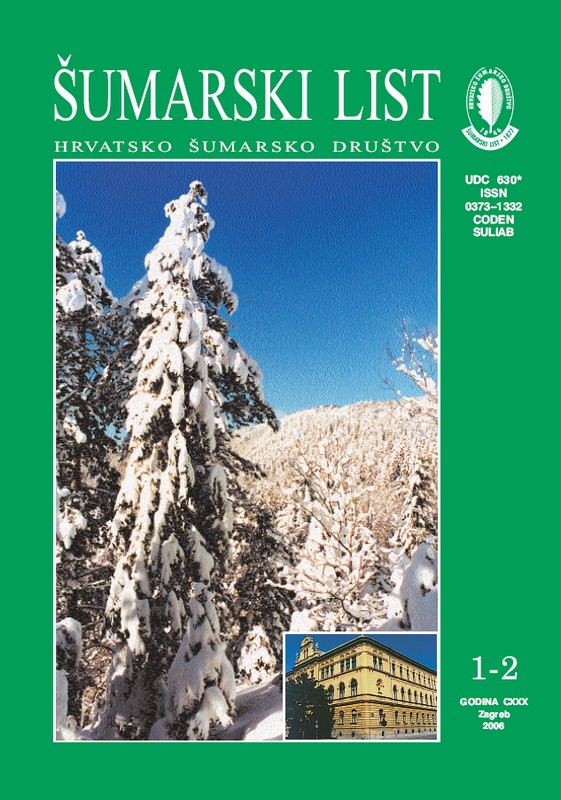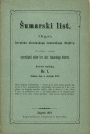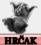broj: 1-2/2006        pdf (19,7 MB)

HR EN

stari brojevi      novi broj

 Znanstveno-stručno i staleško glasilo Hrvatskoga šumarskoga društva Journal of Forestry Society of Croatia Prvi puta izašao 1877. godine i neprekidno izlazi do današnjeg dana ISSN No.: 0373-1332              UDC 630* https://doi.org/10.31298/sl upute autorima WEB EDITION ARHIVA ČASOPISAHRČAKORIGINAL SCIENTIFIC PAPERS Josip Franjić, Željko Škvorc, Andraž Čarni UDK 630* 181.1 + 188 (001) Distribution of Crataegus nigra Waldst. et Kit. in Croatia and its Importance for Forest Edge Vegetation Formation     pdf     HR     EN 3 Juraj Zelić UDK 630* 242 + 651 (001) The Influence of Intensively Thinning on Valuably Shares Of Assortiments Of Meturing Beech Assotiation     pdf     HR     EN 9 Summary: The article compares two plots (plot 1, 2) of normal beech forest (EGT-II-D-10) that are 85 years of age covering 1 ha of surface, in the department 63a, menagement unit “South Papuk”.It was stipulated, from the measured data, the plot 1 was more inensively menaged in the past, that plot 2, but with expertise, which proves the distribution of breast height diameter characteristic for regular stands.Different menagement (more inensive thinning on plot 1, than plot 2) has different effects. On plot 1, there are 346 trees with volume of 452,98 m3/ha, while on plot 2 there are 408 trees with the volume 444,72 m3/ha.Distribution of breast hight diameter and volume per thickness degrees is shown in Table 1.According to earlier menagement type of regular stands, the curve of distribution of breast height diameters is bell-shaped. The corrections of distribution are determined with Beta-functions:F (d) = 0, 007495 S (d - 10) 1,00771 * (65 - d) 1,63964 = 346, for plot 1,F (d) = 0, 002737 S (d - 10) 0,91156 * (65 - d) 2,06635 = 408, for plot 2.The biometrical parameters, as amount of variation of breast height diameters (arithmetical mean, median, first and second exponents of beta-distribution, asymmetry and flatness coeffitient) show how the real distributions of breast height diameters are asymmetrical on the left (positive) side (Table 2).The equaling distribution of volumes per thickness degrees is shown in Graph 1.The structure of asortiments in gross volume per hectare on plots 1. and 2. differentiates as well (Table 4, Graph 2).The value of asortiments in gross volume per hectare shown on Table 5. and Graph 3.It can be concluded that value of wood asortiments per hectare is 8,80 % higher on plot 1, than on plot 2.The research presents a method of calulation of forest interest rate of regular beech stands, that 85 years of age.The basic formula for calculation the current felling value of stands per hectare or volume is: Cn = C0 * 1,0 p n, .The current felling value of regular beech stands per hectare is:Cn = 121113,03 kn/ha,(300,23 kn/m3) for plot 1. and,Cn = 111316,29 kn/ha ( 279,85 kn/m3) for plot 2.The legal standard for biological reproduction is 23 % of actual price of wood assortiments (fcco forest depot).In this case the standards for biological reproduction equals with the costs of establishing forest stands, (C0):C0 = 27856,00 kn/ha, (69,05 kn/m3) for plot 1,C0 = 25602,75 kn/ha (64,36 kn/m3) for plot 2.The forest interest rate (p) is one parameters in the function: The forest interest rate is: p = 1,74 %.The price of stem wood ( forest annuity) is calculated using Barth’s formula:Šn = ( Cn / 1,0 pn) – EnEn, in Barth’s formula represents total costs of exploitation (fco forest depot).The calculated forest annuities (the price of stem wood ) are: Šn = 61,79 kn/m3, for plot 1 and, Šn = 46,43 kn/m3, for plot 2.The price of stem wood (forest annuity) like indicator of menagement economic efficacy is 33,08 % higher on plot 1, than on plot 2.The point of proftability or break-even point (TPn) is 70,40 years for plot 1 and 73,34 years for plot 2 in department 63a, m.u.“South Papuk”.It was affirmed that management economic efficacy of beech stands under mentioned condicions is after 2/3 of beech maturity (100 yeas).Key words: beta-function; breast height diameter distribution; current felling values; forest interest rate (forest annuity); intesivelly thinning; normals; price of stem wood; the asymmetry and flatness coefficients asortiment structure; the point of proftability (break-even point).; Unmixed regular beech forest; value of wood assortiments Sandra Golubić UDK 630* 233 + 114.5 (001) Possibilities of waste disposal on the area of the Međimurje County in the case of the Totovec waste disposal yard     pdf     HR     EN 21 REVIEWS Josip Karavla UDK 630* 272 Dendrological characteristics of the City of Zagreb Green Horseshoe Suggestion for the regeneration of its eastern part     pdf     HR     EN 31 Dalibor Ballian UDK 630* 165 Markers and Basic Mesaures of Genetic Variability Significant in Molecular Genetic Studies     pdf     HR     EN 41 Dario Kremer, Ksenija Karlović, Vesna Židovec UDK 630* 164 Morphometric Research of Natural Populations of Ruscus Hypoglossum L. in Croatia     pdf     HR     EN 47

UNDER CONSTRUCTION